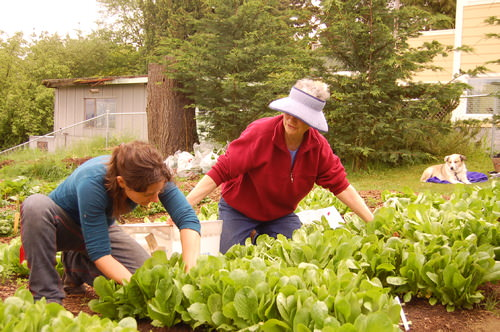# 1.7.3: Simplify Variable Expressions Involving Multiple Operations

•• Contributed by CK12
• CK12

## Simplify Variable Expressions Involving Multiple OperationsFigure $$\PageIndex{1}$$

A farmer, Kelly, has two lovely plots of rectangular land to grow some vegetables. Her friend will help her plant in the spring. One plot of land is 8a by 6a and the other plot of land is 3a by 4a. The two plots of land are going to be combined so they can grow more vegetables. How can Kelly find the total area for both plots of farmable land?

In this concept, you will learn to simplify variable expressions involving multiple operations.

### Simplifying Variable Expressions Involving Multiple Operations

Sometimes, you may need to simplify algebraic expressions that involve more than one operation. Use what you know about simplifying sums, differences, products, or quotients of algebraic expressions to help you do this.

When evaluating expressions, it is important to keep in mind the order of operations, which is

• First, do the computation inside parentheses.
• Second, evaluate any exponents.
• Third, multiply and divide in order from left to right.
• Finally, add and subtract in order from left to right.

Now let’s look at an example.

Simplify this expression 7n+8n⋅3

First, simplify according to the order of operations. According to the order of operations, you should multiply first.

7n+8n⋅3=7n+24n.

7n+24n=31n

Here is another example.

Simplify the expression 10p−7p+8p÷2p.

First, follow the order of operations and rewrite the division as a fraction.

8p/2p

Next, simplify the fraction, assuming p is not equal to zero.

8p/2p=8/2×p/p=4×1=4

Next, rewrite the equation.

10p−7p+(8p/2p)=10p−7p+4

Simplify, by combing like terms.

10p−7p+4=3p+4

### Examples

Example $$\PageIndex{1}$$

Earlier, you were given a problem about Kelly and her two plots of land.

One is 8a by 6a and the other is 3a by 4a.

The plots of land need to be combined to find the total area of farmable land.

Solution

First, consider the equation for the area of a rectangle.

Area of a rectangle=length×width

Next, calculate the area of the first rectangular plot of land.

8a×6a=48a2

Then, calculate the area of the second rectangular plot of land.

3a×4a=12a2

Next, write and expression for the total area of the two plots of land.

48a2+12a2

Finally combine like terms.

48a2+12a2=60a2

Example $$\PageIndex{1}$$

Samera has twice as many pets as Amit has. Kyra has 4 times as many pets as Amit has. Let a represent the number of pets Amit has.

1. Write an expression to represent the number of pets Samera has.
2. Write an expression to represent the number of pets Kyra has.
3. Write an expression to represent the number of pets Samera and Kyra have all together.

Solution

Samera has twice as many pets as Amit. Since Amit has a pets, Samera has 2a pets.

Kyra has 4 times as many pets as Amit has. Since Amit has a pets, Kyra has 4a pets.

To find the number of pets Samera and Kyra have “all together,” write an addition expression.

2a+4a

Finally, combine like terms.

2a+4a=6a

Simplify each expression.

Example $$\PageIndex{1}$$

4a+9a−7

Solution

Combine the like terms 4a and 9a.

Example $$\PageIndex{1}$$

(14x/2)+9x

Solution

First, follow the order of operations and do the division first.

(14x/2)+9x=7x+9x

Next, combine like terms.

7x+9x=16x

Example $$\PageIndex{1}$$

6b−2b+5b−8

Solution

Follow the order of operations and perform the addition and subtraction from left to right.

6b−2b+5b−8=9b−8

### Review

Simplify each expression involving multiple operations.

1. 6a+4a−2b
2. 16b−4b⋅2
3. 22a÷2+14a
4. 19x−5x⋅2
5. 16y−12y÷2
6. 16a−4a−12b
7. 26a+14a+12b+2b
8. 36a+4a−2b+5b
9. 18a+4a+12y
10. 46a+34a−12b+14b
11. 16y+4y−2x
12. 6x+4x+2x+4y−19z
13. 26y−12y÷2
14. 36y−12y÷12
15. 46y+12y÷2

### Vocabulary

Term Definition
Difference The result of a subtraction operation is called a difference.
Expression An expression is a mathematical phrase containing variables, operations and/or numbers. Expressions do not include comparative operators such as equal signs or inequality symbols.
Product The product is the result after two amounts have been multiplied.
Quotient The quotient is the result after two amounts have been divided.
Simplify To simplify means to rewrite an expression to make it as "simple" as possible. You can simplify by removing parentheses, combining like terms, or reducing fractions.
Sum The sum is the result after two or more amounts have been added together.